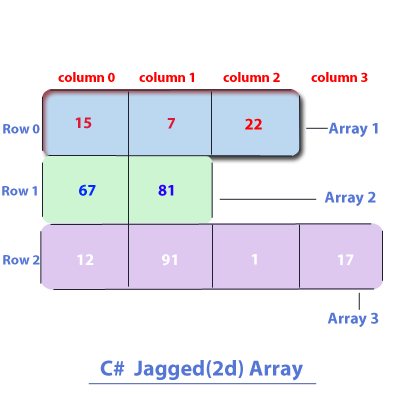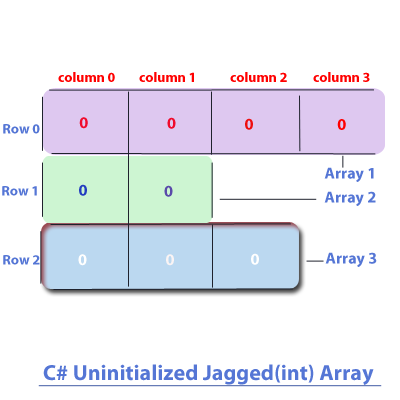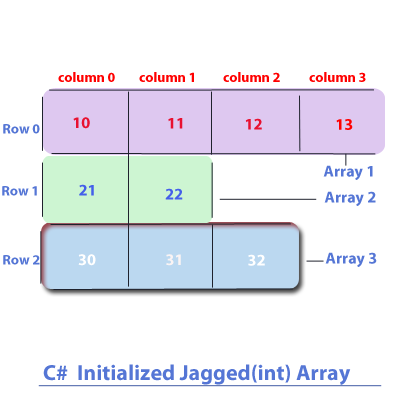Next >

# C# Jagged Array

In one of our previous C# tutorials, we have explained the concept of a 2D array which consists of multiple 1D arrays, where all of the including 1D arrays have equal number of elements, which gives a rectangular/square shape to a 2D array. In this tutorial, we are going to understand jagged array, which is another form of a 2D array.

## What is jagged array?

A jagged array is also a 2D array which is jagged in shape instead of a rectangular/square shape of a general 2D array. Multiple 1D arrays within a 2D jagged array may not have equal number of the elements, which gives a jagged array a jagged shape.

The multiple 1D arrays contained in a 2D jagged array are arranged in rows and columns, where each element of an individual 1D array is accessed by using two index/dimension values:
• First index/dimension value represents a specific row of an element.
• Second index/dimension value represents a specific column of an element.

Let us show you a pictorial representation of a 2D jagged array.## What can a 2D jagged array hold?

A two-dimensional jagged array contains 1D arrays that could hold -
• Primitive values of the same type, or
• Object references of the same type.

## Understanding a 2D jagged array in three parts -

• Declaring a 2D jagged array.
• Constructing a 2D jagged array.
• Initializing a 2D jagged array.

## Note:

Like a 1D array, 2D Array, 3D or an array of any dimension, a jagged array is also an object stored on the Heap memory.

## Declaration of a two-dimensional Jagged array

The general form of declaring a 2D jagged array is :

``type[][] array-name;``
where:
• type is the type of elements this 2D jagged array will hold, followed by sets of empty square brackets [ ][ ] to indicate each dimension i.e row and column.
• array-name, is the name of the array.

An example of declaring a two-dimensional jagged array:
``````//C# Example of two-dimensional jagged array

using System;

class A
{
public static void Main()
{
//Declaration of a 2-D jagged array, which will hold multiple 1D arrays of int values.
int[][] twoDA1;

//Declaring a 2-D jagged array holding 1D arrays of object references of the type class A.
A[][] twoDA2;
}
}``````

Program Analysis: The two 2D jagged array in our example is only declared, hence neither of its incljuding 2D arrays(twoDA1 and twoDA2) exist on the heap yet, and they don't contain any values.

## Construction of a two-dimensional array

The general form of declaring and constructing a two-dimensional array is :

``type[][] array-name = new type[first-dimension][]``
where:
• type is the type of elements this array will hold, followed by sets of empty square brackets [ ][ ].
• array-name is the name of the array.
• new is the keyword using which an array is constructed and gets the memory allocation.
• first-dimension represents the total number of 1D arrays contained in this 2D jagged array and this is followed by a set of empty square brackets [ ].

• Note: The second set of square brackets is left empty because it should represent the total number of elements in each 1D array contained in a 2D array, and because each 1D array in this 2D jagged array may have different number of elements, so we don't need to specify any value in the second set of square brackets.

An Example of constructing a two-dimensional jagged array.
``````//C# Example of constructing a two-dimensional array

using System;

class A
{
public static void Main()
{
//A 2D jagged Array is constructed on the heap with a size to hold 3 1D int arrays.
//Where, we are still to define the total number of elements in each of these 1D arrays.
int[][] twoDA1= new int[];

//First 1D array will have 4 int values
twoDA1 = new int;

//Second 1D array will have 2 int values
twoDA1 = new int;

//Third 1D array will have 3 int values
twoDA1 = new int;

//Prints the total number of 1D array contains in this 2D jagged array
Console.WriteLine("Total number of 1D arrays in a 2D jagged array :" + twoDA1.Length);

//Reading the elements of the first 1D array by using its index 0
Console.WriteLine("Reading the {0} elements of the first 1D array:", twoDA1.Length);
for(int i=0; i<4; i++)
Console.WriteLine(twoDA1[i]);

//Reading the elements of the second 1D array by using its index 1
Console.WriteLine("Reading the {0} elements of the second 1D array:", twoDA1.Length);
for(int i=0; i<2; i++)
Console.WriteLine(twoDA1[i]);

//Reading the elements of the third 1D array by using its index 2
Console.WriteLine("Reading the {0} elements of the third 1D array:", twoDA1.Length);
for(int i=0; i<3; i++)
Console.WriteLine(twoDA1[i]);

}
}``````

Output
``````Total number of 1D arrays in a 2D jagged array :3
Reading the 4 elements of the first 1D array:
0
0
0
0
Reading the 2 elements of the second 1D array:
0
0
Reading the 3 elements of the third 1D array:
0
0
0``````

Program Analysis: In our last example, the 2D jagged array(which contains 1D arrays of numeric primitive values) is not initialized with values, therefore, the elements of each of its 1D arrays are automatically initialized with zero values.

In the example, we have also accessed each of the three int 1D arrays using its index position in the 2D jagged array, where:
• The reference to the first array is specified by its index i.e. 0.
• The reference to the second array is specified by its index i.e. 1.
• The reference to the third array is specified by its index i.e. 2.

Post the construction of our 2D jagged array, it is depicted in the diagram below.As these 3 int arrays are only constructed, and we have not manually initialized their elements yet, therefore, they are initialized with their default values:
• An array holding any numeric primitive type values will be automatically initialized to zero(0), and,
• An array holding object references will be automatically initialized to null.
Please look at the table to know more about the default values gives to an array(if not initialized with values) -

Array type Default value
char '\u0000'
byte 0
short 0
int 0
long 0
float 0
double 0
bool false
String null
Object null

## Initialization and Reading of a two-dimensional array

Our 2D int jagged array created in the last example contains three 1D arrays, with their elements automatically initialized to zeroes. But let's manually initialize elements of the three 1D arrays with int values.

``````//C# Example of initializing a two-dimensional array

using System;

class A
{
public static void Main()
{
//A 2D jagged Array is constructed on the heap with a size to hold 3 1D int arrays.
//Where, we are still to define the total number of elements in each of these 1D arrays.
int[][] twoDA1= new int[];

//First 1D array will have 4 int values
twoDA1 = new int;

//Second 1D array will have 2 int values
twoDA1 = new int;

//Third 1D array will have 3 int values
twoDA1 = new int;

//Prints the total number of 1D array contains in this 2D jagged array
Console.WriteLine("Total number of 1D arrays in a 2D jagged array :" + twoDA1.Length);

//Initializing the elements of the first 1D array by using its index 0
Console.WriteLine("Initializing the {0} elements of the first 1D array ", twoDA1.Length);
for(int i=0; i<4; i++)
twoDA1[i] = i + 10;

//Initializing the elements of the second 1D array by using its index 1
Console.WriteLine("Initializing the {0} elements of the second 1D array ", twoDA1.Length);
for(int i=0; i<2; i++)
twoDA1[i] = i + 20;

//Initializing the elements of the third 1D array by using its index 2
Console.WriteLine("Initializing the {0} elements of the third 1D array", twoDA1.Length);
for(int i=0; i<3; i++)
twoDA1[i] = i + 30;

//Reading the elements of the first 1D array by using its index 0
Console.WriteLine("Reading the {0} elements of the first 1D array:", twoDA1.Length);
for(int i=0; i<4; i++)
Console.WriteLine(twoDA1[i]);

//Reading the elements of the second 1D array by using its index 1
Console.WriteLine("Reading the {0} elements of the second 1D array:", twoDA1.Length);
for(int i=0; i<2; i++)
Console.WriteLine(twoDA1[i]);

//Reading the elements of the third 1D array by using its index 2
Console.WriteLine("Reading the {0} elements of the third 1D array:", twoDA1.Length);
for(int i=0; i<3; i++)
Console.WriteLine(twoDA1[i]);

}
}
``````

Output:
``````Total number of 1D arrays in a 2D jagged array :3
Initializing the 4 elements of the first 1D array
Initializing the 2 elements of the second 1D array
Initializing the 3 elements of the third 1D array
Reading the 4 elements of the first 1D array:
10
11
12
13
Reading the 2 elements of the second 1D array:
21
22
Reading the 3 elements of the third 1D array:
30
31
32``````
Depiction of the initializing the 2D jagged array is shown in the picture below.## A 2D Jagged array holding 1D arrays of object references

A 2D jagged array can also hold 1D arrays that are holding object references of the same type. Let's see an example -

``````// C# 2-D Jagged Array holding arrays of object references

using System;

class A
{
public static void Main()
{
//A 2D jagged Array is constructed on the heap with a size to hold 3 1D arrays to hold object references of A.
//But, we are still to define the total number of object references/elements in each of these 1D arrays.
A[][] twoDA1= new A[];

//First 1D array will have 4 object references of A
twoDA1 = new A;

//Second 1D array will have 2 object references of A
twoDA1 = new A;

//Third 1D array will have 3 object references of A
twoDA1 = new A;

//Prints the total number of 1D array contains in this 2D jagged array
Console.WriteLine("Total number of 1D arrays in a 2D jagged array :" + twoDA1.Length);

//Initializing the elements of the first 1D array by using its index 0
Console.WriteLine("Initializing the {0} elements of the first 1D array ", twoDA1.Length);
for(int i=0; i<4; i++)
twoDA1[i] = new A();

//Initializing the elements of the second 1D array by using its index 1
Console.WriteLine("Initializing the {0} elements of the second 1D array ", twoDA1.Length);
for(int i=0; i<2; i++)
twoDA1[i] = new A();

//Initializing the elements of the third 1D array by using its index 2
Console.WriteLine("Initializing the {0} elements of the third 1D array", twoDA1.Length);
for(int i=0; i<3; i++)
twoDA1[i] = new A();

//Prints the total number of 1D array contains in this 2D jagged array
Console.WriteLine("Total number of 1D arrays in a 2D jagged array :" + twoDA1.Length);

//Reading the elements of the second 1D array by using its index 0
Console.WriteLine("Reading the {0} elements of the first 1D array:", twoDA1.Length);
for(int i=0; i<4; i++)
Console.WriteLine(twoDA1[i]);

//Reading the elements of the second 1D array by using its index 1
Console.WriteLine("Reading the {0} elements of the second 1D array:", twoDA1.Length);
for(int i=0; i<2; i++)
Console.WriteLine(twoDA1[i]);

//Reading the elements of the third 1D array by using its index 2
Console.WriteLine("Reading the {0} elements of the third 1D array:", twoDA1.Length);
for(int i=0; i<3; i++)
Console.WriteLine(twoDA1[i]);

}
}``````

## Output-

``````Total number of 1D arrays in a 2D jagged array :3
Initializing the 4 elements of the first 1D array
Initializing the 2 elements of the second 1D array
Initializing the 3 elements of the third 1D array
Total number of 1D arrays in a 2D jagged array :3
Reading the 4 elements of the first 1D array:
A
A
A
A
Reading the 2 elements of the second 1D array:
A
A
Reading the 3 elements of the third 1D array:
A
A
A``````

Program Analysis: In the last example, we have created a 2D jagged array to hold three 1D arrays, with each 1D array holding different number of object references to objects of type A. Next, we have initialized each element the contained 1D arrays to an object of type A.

## A 2D jagged array holding object references will be automatically initialized to null

Unlike the last example of a jagged array, if the elements of its each 1D array(holding object references) are not initialized, then the elements will be automatically initialized to null.

``````//C# A 2D jagged array holding object references will be automatically initialized to null

using System;

class A
{
public static void Main()
{
//A 2D jagged Array is constructed on the heap with a size to hold 3 1D arrays to hold object references of A.
//But, we are still to define the total number of object references/elements in each of these 1D arrays.
A[][] twoDA1= new A[];

//First 1D array will have 4 object references of A
twoDA1 = new A;

//Second 1D array will have 2 object references of A
twoDA1 = new A;

//Third 1D array will have 3 object references of A
twoDA1 = new A;

//Prints the total number of 1D array contains in this 2D jagged array
Console.WriteLine("Total number of 1D arrays in a 2D jagged array :" + twoDA1.Length);

//Reading the elements of the second 1D array by using its index 0
Console.WriteLine("Reading the {0} elements of the uninitialized first 1D array:", twoDA1.Length);
for(int i=0; i<4; i++)
{
if(twoDA1[i]==null)
Console.WriteLine(twoDA1[i] + "A null element");
}

//Reading the elements of the second 1D array by using its index 1
Console.WriteLine("Reading the {0} elements of the uninitialized second 1D array:", twoDA1.Length);
for(int i=0; i<2; i++)
{
if(twoDA1[i]==null)
Console.WriteLine(twoDA1[i] + "A null element");
}

//Reading the elements of the third 1D array by using its index 2
Console.WriteLine("Reading the {0} elements of the uninitialized third 1D array:", twoDA1.Length);
for(int i=0; i<3; i++)
{
if(twoDA1[i]==null)
Console.WriteLine(twoDA1[i] + "A null element");
}

}
}``````

## Output:

``````Total number of 1D arrays in a 2D jagged array :3
Reading the 4 elements of the uninitialized first 1D array:
A null element
A null element
A null element
A null element
Reading the 2 elements of the uninitialized second 1D array:
A null element
A null element
Reading the 3 elements of the uninitialized third 1D array:
A null element
A null element
A null element
``````

In the last example, a 2D jagged array to hold three 1D arrays of type A is constructed on the heap, but the elements of these 1D arrays are not manually initialized by us, therefore, the elements of these 1D arrays will be automatically initialized to null i.e. a reference points to no value. Hence, nothing is printed when the null is printed.

Please subscribe to our social media channels for daily updates.